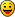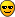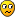## Transversals in Sudoku Squares

Everything about Sudoku that doesn't fit in one of the other sections

### Re: Transversals in Sudoku Squares

Hi, people!
Here are some new search results.
1000000 random P-sudokus were checked - has it at least one "Afghan" pair (i.e. is it possible to buld "Afghan grid" from checked P-sudoku and its pair)? I got 1729 "Afghan-ready" P-sudokus (ratio 1:600 approx.). Then I analyzed those 1729 "Afghan-ready" P-sudokus.

It is known, that any band (stack) of sudoku solution grid may contain "repeating triples" or not. So, there are 2 possible types of any band (stack). I counted bands and stacks containing "repeating triples". For example, MC grid has 3 bands containing "repeating triples" and 3 stacks containing "repeating triples". So, MC grid would have such count equal to 6.

Some statistics concerning "repeating triples" of 1729 "Afghan-ready" P-sudokus.
Code: Select all
`Number of bands and stacks with triples   Grids                                      0      67                                      1     289                                      2     534                                      3     532                                      4     247                                      5      53                                      6       7`

P-sudokus without "repeating triples" are more rare, than one would expect (67 grids), but P-sudokus having all bands and stack with "repeating triples" (like MC grid) are more frequent, than one would expect (7 grids).

If a band is randomly generated, than probability of "repeating triples" appearance is 1/4. Probability of having 3 bands and 3 stacks containing "repeating triples" - (1/4)^6 = 0.000244, that corresponds to 0.4 grids among 1729 grids (20 time less, than observed). On the other hand, probability of having all bands and stacks without "repeating triples" - (3/4)^6 = 0.178, that corresponds to 307.7 grids among 1729 grids (5 times higher than observed).

So, more bands/stacks with "repeating triples" are there, higher probability of random P-sudoku to be "Afghan ready" is.

Serg
Serg
2018 Supporter

Posts: 690
Joined: 01 June 2010
Location: Russia

### Re: Transversals in Sudoku Squares

That's strange! I use either notepad or MS Word 2010 and the dagger mark † pastes correctly into the forum's reply window.

the ASCII code table in windows 10 is different then all previous generations of windows. they used to be three digit code for extended characters most of the tables were updated with a 4 digit sequence
a lot of software never updated to the new differences and fail to display that item specifically when copied and pasted over.

some stuff still works as it uses different coding tables like HTML browsers.

i have this problem with my solver program it fails to translate my display function correctly on newer windows.... my txt appears correct in my editor but goes garbled in windows 10.... but fine on my win98,2000,xp,vista, 7,8 systems.

to find the new map code in win10
search for charmap in the search bar and it should popup...

also character font type also throws a wrench into what can be displayed as well. {some character maps are not coded into its data base}
Some do, some teach, the rest look it up.StrmCkr

Posts: 1085
Joined: 05 September 2006

### Re: Transversals in Sudoku Squares

This is a dagger:

It actually works, it's just that the font used by my trusty old text editor (PFE, 1998) doesn't have an entry for that Ascii code, so it just shows as a solid blob, but copies/pastes correctly as can be seen here.Mathimagics
2017 Supporter

Posts: 1480
Joined: 27 May 2015
Location: Canberra

### Re: Transversals in Sudoku Squares

a better code writing tool{just figured id chime in what i know about the code table as it was a pain to work around....when i upgraded to win10...}

my last comment was correct in that case i guessSome do, some teach, the rest look it up.StrmCkr

Posts: 1085
Joined: 05 September 2006

### Re: Transversals in Sudoku Squares

Getting back on topic, does anybody know if gsf's Sudoku tool (or any other tool) has an option to generate all 3,359,232 geometric transformations of a grid?

That would be a way of double-checking that the counter-example I posted above is indeed not transformable to SudokuP form.Mathimagics
2017 Supporter

Posts: 1480
Joined: 27 May 2015
Location: Canberra

### Re: Transversals in Sudoku Squares

Mathemagics, is it possible to rename "SudokuP" to "P-Sudoku"? I think, it is more convenient - it would be possible to write this term in plural form and relation between "P-set" and "P-Sudoku" will be more evident

Ok, I will start using P-Sudoku .....

...................... if you start using MathimagicsMathimagics
2017 Supporter

Posts: 1480
Joined: 27 May 2015
Location: Canberra

### Re: Transversals in Sudoku Squares

transpose =(2)
row =(3!)^3
col =(3!)^3
band= 3!
stack =3!
== 2(3!^8)
=> 3,359,232

my program can, but atm it only does it by user selection 1 by 1.
wouldn't take much for me to convert it to automated to do all of them

my code snippet... if you want to write your own version {this is in freepascal turbo pascal dialect}
Hidden Text: Show
Code: Select all
`procedure issomorph(K2,B2,F2:char);varK,B,F:char;xn,n:integer;S2:  array [cell] of nums;  {solved grid copy}beginfor xn:= 0 to 80 do s2[xn]:=S[xn];for xn:= 0 to 8 do  begin   Rd[xn]:= [1..9];   Cd[xn]:= [1..9];   Bd[xn]:= [1..9];   covered2[xn+1]:=[0..80];    for n:= 1 to 9 do      begin      // S[rset[xn,(n-1)]]:= [];       delpm[rset[xn,(n-1)]]:= [1..9];       covered[rset[xn,(n-1)]]:= [1..9];      end;   end;k:=k2;b:=b2;f:=f2;if not (K in ['d','t','s','b','c','r']) then   beginwritexy(2,25,' which area to move, R{ow},C{ol},s{tack},B{and},T{ranspose},D{igit}');K:=readkey;  write(': ',K);   end; case K of{R} #114: begin   if not (B in ['1'..'9'])    then     begin   writexy(2,26,' Which Row to move? {1..9}');   B:=readkey;  write(': ',B);     end;   if not (F in ['1'..'9'])    then     begin   writexy(2,27,' Swaping with Row?');   case B of    #49,#50,#51 : write(' 1,2,3');    #52,#54,#55 : write(' 4,5,6');    #56,#57,#58 : write(' 7,8,9');   end;   F:=readkey; write(': ',F);    end;   for xn:=0 to 8 do    begin    s[Rset[(ord(b)-48)-1,xn]]:= S2[Rset[(ord(f)-48)-1,xn]];    s[Rset[(ord(f)-48)-1,xn]]:= S2[Rset[(ord(b)-48)-1,xn]];    end;  end;  {row}{C} #99: begin  if not (B in ['1'..'9'])    then     begin   writexy(2,26,' Which Col to move? {1..9}');   B:=readkey;  write(': ',B);     end;   if not (F in ['1'..'9'])    then     begin   writexy(2,27,' Swaping with Col?');   case B of    #49,#50,#51 : write(' 1,2,3');    #52,#54,#55 : write(' 4,5,6');    #56,#57,#58 : write(' 7,8,9');   end;   F:=readkey; write(': ',F);    end;   for xn:=0 to 8 do    begin    s[Cset[(ord(b)-48)-1,xn]]:= S2[Cset[(ord(f)-48)-1,xn]];    s[Cset[(ord(f)-48)-1,xn]]:= S2[Cset[(ord(b)-48)-1,xn]];    end; end; {col}{Band} #98: begin    if not (B in ['1'..'3'])    then     begin   writexy(2,26,' Which Band to Swap? {1..3}');   B:=readkey;  write(': ',B);     end;    if not (F in ['1'..'3'])    then     begin   writexy(2,27,' Swaping with Band? {1..3}');   F:=readkey;  write(': ',F);      end;   For xn:= 0 to 8 do    for n:= 0 to 2 do      begin        s[Rset[(((ord(b)-48)-1)*3+n),xn]]:=s2[Rset[(((ord(f)-48)-1)*3+n),xn]];        s[Rset[(((ord(f)-48)-1)*3+n),xn]]:=s2[Rset[(((ord(b)-48)-1)*3+n),xn]];      end; end;  {Band}{stack} #115: begin     if not (B in ['1'..'3'])    then     begin   writexy(2,26,' Which Stack to Swap? {1..3}');   B:=readkey;  write(': ',B);     end;    if not (F in ['1'..'3'])    then     begin   writexy(2,27,' Swaping with Stack? {1..3}');   F:=readkey;  write(': ',F);      end;   For xn:= 0 to 8 do    for n:= 0 to 2 do      begin        s[Cset[(((ord(b)-48)-1)*3+n),xn]]:=s2[Cset[(((ord(f)-48)-1)*3+n),xn]];        s[Cset[(((ord(f)-48)-1)*3+n),xn]]:=s2[Cset[(((ord(b)-48)-1)*3+n),xn]];      end; end; {stack}{Transpose} #116: begin if not(k2 in['t']) then writexy(2,26,' Transposing Grid (main diagonal reflection)'); for xn:= 0 to 8 do  for n:= 0 to 8 do   begin     S[Rset[xn,n]]:=s2[Cset[xn,n]];     s[Cset[n,xn]]:=S2[Rset[n,xn]];    end; end; {Transpose}{D} #100: begin  if not (b in ['1'..'9'] )   then   begin  writexy(2,26,' Which Digit to Swap? {1..9}');   B:=readkey;  write(': ',B);   end;  if not (f in ['1'..'9'] )   then   begin   writexy(2,27,' Swaping with Digit {1..9}');   F:=readkey;  write(': ',F);    end;   For xn:= 0 to 80 do   begin    if S2[xn]= [ord(b)-48 ]      then      s[xn]:=[ord(F)-48];    if S2[xn]= [ord(F)-48 ]      then      s[xn]:=[ord(B)-48];    end; end;   end; setpm; Sbrc; wpm(1);writexy(2,25,'                                                                      ');writexy(2,26,'                                                                      ');writexy(2,27,'                                                                      ');end;  {issomorphic transformation`

Code: Select all
`That would be a way of double-checking that the counter-example I posted above is indeed not transformable to SudokuP form.`

{ if i recall correctly}
a short cut method would be to solve both grids to completion
do a digit swap on row 1 so it reads
123456789
do the same to the 2nd puzzle,

if both of them are isomorphic their solution is identical

with out having to go through all the permutations of the above
as digits swap are replicated by transformations.
Some do, some teach, the rest look it up.StrmCkr

Posts: 1085
Joined: 05 September 2006

### Re: Transversals in Sudoku Squares

The more I look at the more I trust my results, only 6^6 transformations affect P-sets, and I have correctly applied them, so that grid was certainly not transformable to P-Sudoku.

But thanks anyway!Agree that's the best way to compare two solutions. The case in point is a different one, though - given one grid which is not P-Sudoku, is there an isomorph that IS P-Sudoku?Mathimagics
2017 Supporter

Posts: 1480
Joined: 27 May 2015
Location: Canberra

### Re: Transversals in Sudoku Squares

Silly me, I never thought to check eleven's example above, which DOES have a SudokuP (whoops, P-Sudoku) isomorph.

My test function correctly identified it.Mathimagics
2017 Supporter

Posts: 1480
Joined: 27 May 2015
Location: Canberra

### Re: Transversals in Sudoku Squares

All puzzles have at least 5 clues out of 9 "transversal" clue pattern
There are only 2 non-isomorphic 17-puzzles with a complete 9 clue transversal [ its easy to understand why there is only 2 ]
around 50% of 17-puzzles have 7 clues from a transversal [ there are 2 ways to have 7 clues]
It didnt turn out very fruitful way to find 17-puzzles !
coloin

Posts: 1861
Joined: 05 May 2005

### Re: Transversals in Sudoku Squares

Is a SudokuP grid just another name for a Sudoku DG grid ?
Where there are 9 additional groups with clues 1-9
Code: Select all
`. x . | . x . | . x .. . . | . . . | . . .. . . | . . . | . . .---------------------. x . | . x . | . x .. . . | . . . | . . .. . . | . . . | . . .---------------------. x . | . x . | . x .. . . | . . . | . . .. . . | . . . | . . . `
coloin

Posts: 1861
Joined: 05 May 2005

### Re: Transversals in Sudoku Squares

Yes coloin, the 9 groups with the same position in each box.

Mathimagics wrote:In response to eleven's question about whether a non-P grid can be made into a SudokuP grid by row/col permutations, I think this one shows this is not always the case:
...

I never expected, that this could be done for arbitrary grids. Suppose you have managed to find a constellation with the first minirow fitting. Then all you can do is swapping rows 2 and 3 in each band.
[Edit: my conclusion was rubbish. If you manage do make rows 258, you are finished - the rest are 3 digits for each column, which always must fit for a PSudoku]
My conjecture was just for puzzles with minirow symmetry (and probably any other symmetry with 3-cycles).
eleven

Posts: 2241
Joined: 10 February 2008

### Re: Transversals in Sudoku Squares

coloin wrote:Is a SudokuP grid just another name for a Sudoku DG grid ?

Yes it is. Oh dear, yet ANOTHER name for SudokuP !!!DG - "Disjoint groups"? It's a little clunky ...

But I note with interest that Ocean came up with 11 clue SudokuP examples.

Is this the absolute minimum? That's got to be hard to beat ...Mathimagics
2017 Supporter

Posts: 1480
Joined: 27 May 2015
Location: Canberra

### Re: Transversals in Sudoku Squares

Mathimagics wrote:
coloin wrote:Is a SudokuP grid just another name for a Sudoku DG grid ?

Yes it is. Oh dear, yet ANOTHER name for SudokuP !!!"Also known as Offset Sudoku" , Alias: Color Sudoku, Farbsudoku, 4D Sudoku, Plum Sudoku, also the Even-odd Sudokueleven

Posts: 2241
Joined: 10 February 2008

### Re: Transversals in Sudoku Squares

Here Red Ed posts the number of disjoint group sudokus as being 201105135151764480.
This gives a rate of 1:33171
eleven

Posts: 2241
Joined: 10 February 2008

PreviousNext